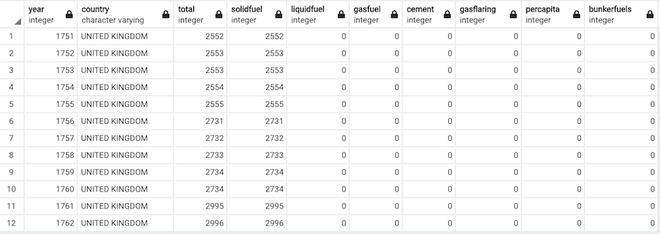How to insert a pandas DataFrame to an existing PostgreSQL table?

• Last Updated : 22 Nov, 2021

In this article, we are going to see how to insert a pandas DataFrame to an existing PostgreSQL table.

Modules needed

• pandas: Pandas DataFrame is two-dimensional size-mutable, potentially heterogeneous tabular data structure with labeled axes (rows and columns). A Data frame is a two-dimensional data structure, i.e., data is aligned in a tabular fashion in rows and columns. Pandas DataFrame consists of three principal components, the data, rows, and columns.
• psycopg2: PostgreSQL is a powerful, open source object-relational database system. PostgreSQL runs on all major operating systems. PostgreSQL follows ACID property of DataBase system and has the support of triggers, updatable views and materialized views, foreign keys.
• sqlalchemy: SQLAlchemy is the Python SQL toolkit and Object Relational Mapper that gives application developers the full power and flexibility of SQL

we start the code by importing packages and creating a connection string of the format:

The create_engine() function takes the connection string as an argument and forms a connection to the PostgreSQL database, after connecting we create a dictionary, and further convert it into a dataframe using the method pandas.DataFrame() method.

The to_sql() method is used to insert a  pandas data frame into the Postgresql table. Finally, we execute commands using the execute() method to execute our SQL commands and fetchall() method to fetch the records.

df.to_sql(‘data’, con=conn, if_exists=’replace’, index=False)

arguments are:

• name of the table
• connection
• if_exists : if the table already exists the function we want to apply . ex: ‘append’ help us add data instead of replacing the data.
• index : True or False

Example 1:

Insert a pandas DataFrame to an existing PostgreSQL table using sqlalchemy. The create table command used to create a table in the PostgreSQL database in the following example  is:

create table data( Name varchar, Age bigint);

Code:

Python3

 import psycopg2import pandas as pdfrom sqlalchemy import create_engine    conn_string = 'postgres://user:password@host/data1'  db = create_engine(conn_string)conn = db.connect()    # our dataframedata = {'Name': ['Tom', 'dick', 'harry'],        'Age': [22, 21, 24]}  # Create DataFramedf = pd.DataFrame(data)df.to_sql('data', con=conn, if_exists='replace',          index=False)conn = psycopg2.connect(conn_string                        )conn.autocommit = Truecursor = conn.cursor()  sql1 = '''select * from data;'''cursor.execute(sql1)for i in cursor.fetchall():    print(i)  # conn.commit()conn.close()

Output:

('Tom', 22)
('dick', 21)
('harry', 24)

Output in PostgreSQL:output table in PostgreSQL

Example 2:

Insert a pandas DataFrame to an existing PostgreSQL table without using sqlalchemy. As usual, we form a connection to PostgreSQL using the connect() command and execute the execute_values() method, where there’s the ‘insert’ SQL command is executed. a try-except clause is included to make sure the errors are caught if any.

The create table command used to create a table in the PostgreSQL database in the following example is :

create table fossil_fuels_c02(year int, country varchar,total int,solidfuel int, liquidfuel int,gasfuel int,cement int,gasflaring int,percapita int,bunkerfuels int);

Code:

Python3

 import psycopg2import numpy as npimport psycopg2.extras as extrasimport pandas as pd    def execute_values(conn, df, table):      tuples = [tuple(x) for x in df.to_numpy()]      cols = ','.join(list(df.columns))    # SQL query to execute    query = "INSERT INTO %s(%s) VALUES %%s" % (table, cols)    cursor = conn.cursor()    try:        extras.execute_values(cursor, query, tuples)        conn.commit()    except (Exception, psycopg2.DatabaseError) as error:        print("Error: %s" % error)        conn.rollback()        cursor.close()        return 1    print("the dataframe is inserted")    cursor.close()    conn = psycopg2.connect(    database="ENVIRONMENT_DATABASE", user='postgres', password='pass', host='127.0.0.1', port='5432')  df = pd.read_csv('fossilfuels.csv')  execute_values(conn, df, 'fossil_fuels_c02')

Output:

the dataframe is insertedafter inserting the dataFrame

My Personal Notes arrow_drop_up